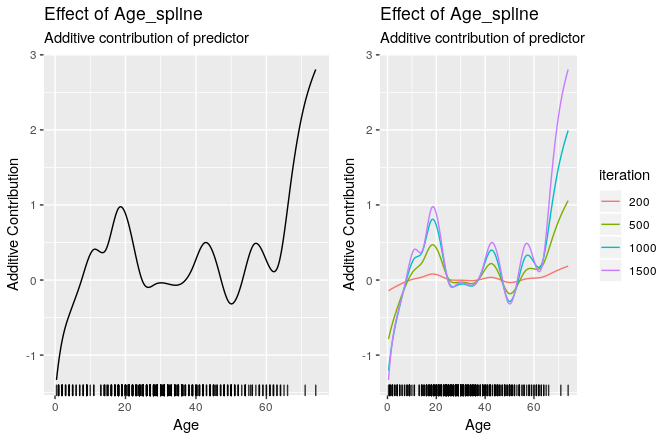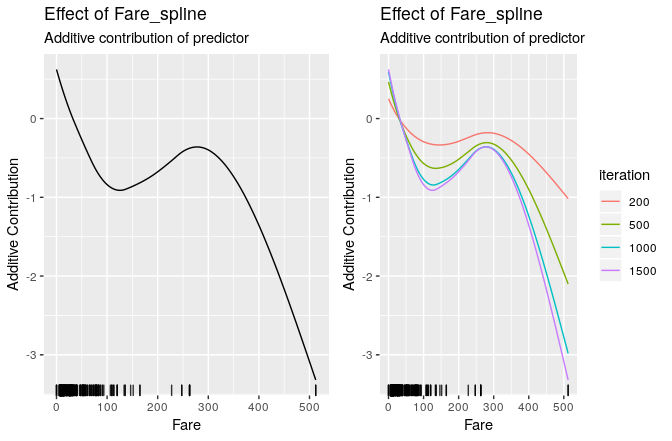# compboost: Fast and Flexible Component-Wise Boosting Framework

## Data: Titanic Passenger Survival Data Set

We use the titanic dataset with binary classification on survived. First of all we store the train and test data in two data frames and remove all rows that contains NAs:

# Store train and test data:
df.train = na.omit(titanic::titanic_train)
df.test  = na.omit(titanic::titanic_test)

str(df.train)
## 'data.frame':    714 obs. of  12 variables:
##  $PassengerId: int 1 2 3 4 5 7 8 9 10 11 ... ##$ Survived   : int  0 1 1 1 0 0 0 1 1 1 ...
##  $Pclass : int 3 1 3 1 3 1 3 3 2 3 ... ##$ Name       : chr  "Braund, Mr. Owen Harris" "Cumings, Mrs. John Bradley (Florence Briggs Thayer)" "Heikkinen, Miss. Laina" "Futrelle, Mrs. Jacques Heath (Lily May Peel)" ...
##  $Sex : chr "male" "female" "female" "female" ... ##$ Age        : num  22 38 26 35 35 54 2 27 14 4 ...
##  $SibSp : int 1 1 0 1 0 0 3 0 1 1 ... ##$ Parch      : int  0 0 0 0 0 0 1 2 0 1 ...
##  $Ticket : chr "A/5 21171" "PC 17599" "STON/O2. 3101282" "113803" ... ##$ Fare       : num  7.25 71.28 7.92 53.1 8.05 ...
##  $Cabin : chr "" "C85" "" "C123" ... ##$ Embarked   : chr  "S" "C" "S" "S" ...
##  - attr(*, "na.action")= 'omit' Named int  6 18 20 27 29 30 32 33 37 43 ...
##   ..- attr(*, "names")= chr  "6" "18" "20" "27" ...

In the next step we transform the response to a factor with more intuitive levels:

df.train$Survived = factor(df.train$Survived, labels = c("no", "yes"))

# Train and evaluation split for training:
set.seed(1111)

idx.train = sample(x = seq_len(nrow(df.train)), size = 0.6 * nrow(df.train))
idx.eval  = setdiff(seq_len(nrow(df.train)), idx.train) 

This split will be used while the training to calculate the out of bag risk.

## Initializing Model

Due to the R6 API it is necessary to create a new class object which gets the data, the target as character, and the used loss. Note that it is important to give an initialized loss object:

cboost = Compboost$new(data = df.train[idx.train, ], target = "Survived", loss = LossBinomial$new())

Use an initialized object for the loss gives the opportunity to use a loss initialized with a custom offset.

Adding new base-learners is also done by giving a character to indicate the feature. As second argument it is important to name an identifier for the factory since we can define multiple base-learner on the same source.

### Numerical Features

For instance, we can define a spline and a linear base-learner of the same feature:

# Spline base-learner of age:
cboost$addBaselearner("Age", "spline", BaselearnerPSpline) # Linear base-learner of age (degree = 1 with intercept is default): cboost$addBaselearner("Age", "linear", BaselearnerPolynomial)

Additional arguments can be specified after naming the base-learner. For a complete list see the functionality at the project page:

# Spline base-learner of fare:
cboost$addBaselearner("Fare", "spline", BaselearnerPSpline, degree = 2, n.knots = 14, penalty = 10, differences = 2) ### Categorical Features When adding categorical features each group is added as single base-learner to avoid biased feature selection. Also note that we don’t need an intercept here: cboost$addBaselearner("Sex", "categorical", BaselearnerPolynomial,
intercept = FALSE)

Finally, we can check what factories are registered:

cboost$getBaselearnerNames() ##  "Age_spline" "Age_linear" "Fare_spline" ##  "Sex_male_categorical" "Sex_female_categorical" ## Define Logger ### Time logger This logger logs the elapsed time. The time unit can be one of microseconds, seconds or minutes. The logger stops if max_time is reached. But we do not use that logger as stopper here: cboost$addLogger(logger = LoggerTime, use.as.stopper = FALSE, logger.id = "time",
max.time = 0, time.unit = "microseconds")

### Out of bag risk logger

The out of bag risk logger does basically the same as the inbag risk logger but calculates the empirical risk using another data source. Therefore, the new data object have to be a list with data sources containing the evaluation data. This is automatically done by the prepareData() member of Compboost:

cboost$addLogger(logger = LoggerOobRisk, use.as.stopper = FALSE, logger.id ="oob", LossBinomial$new(), 0.01, cboost$prepareData(df.train[idx.eval, ]), df.train[["Survived"]][idx.eval]) ## Train Model and Access Elements After defining all object we can train the model: cboost$train(1000, trace = 50)
##    1/1000: risk = 0.67             0.52:                 1:
##   50/1000: risk = 0.61             0.53:              5365:
##  100/1000: risk = 0.57             0.59:             11033:
##  150/1000: risk = 0.55             0.65:             16902:
##  200/1000: risk = 0.54             0.69:             23193:
##  250/1000: risk = 0.52             0.73:             29558:
##  300/1000: risk = 0.52             0.77:             35896:
##  350/1000: risk = 0.51             0.80:             42287:
##  400/1000: risk = 0.51             0.82:             48635:
##  450/1000: risk = 0.5             0.85:             55060:
##  500/1000: risk = 0.5             0.87:             62553:
##  550/1000: risk = 0.5             0.89:             69050:
##  600/1000: risk = 0.5             0.91:             75534:
##  650/1000: risk = 0.49             0.93:             81984:
##  700/1000: risk = 0.49             0.94:             88576:
##  750/1000: risk = 0.49             0.95:             95116:
##  800/1000: risk = 0.49             0.96:            101650:
##  850/1000: risk = 0.49             0.97:            108186:
##  900/1000: risk = 0.49             0.98:            114815:
##  950/1000: risk = 0.49             0.99:            121418:
## 1000/1000: risk = 0.49             1.00:            128096:
##
##
## Train 1000 iterations in 0 Seconds.
## Final risk based on the train set: 0.49
cboost
## Component-Wise Gradient Boosting
##
## Trained on df.train[idx.train, ] with target Survived
## Number of base-learners: 5
## Learning rate: 0.05
## Iterations: 1000
## Positive class: no
## Offset: 0.2529
##
## LossBinomial Loss:
##
##   Loss function: L(y,x) = log(1 + exp(-2yf(x))
##
## 

## Accessing Elements

Object of the Compboost class do have member functions such as getEstimatedCoef(), getInbagRisk() or predict() to access the results:

str(cboost$getEstimatedCoef()) ## List of 5 ##$ Age_spline            : num [1:24, 1] -2.5721 -1.0457 -0.4804 -0.0319 0.3728 ...
##  $Fare_spline : num [1:17, 1] 0.969 0.215 -0.291 -0.704 -0.864 ... ##$ Sex_female_categorical: num [1, 1] -1.33
##  $Sex_male_categorical : num [1, 1] 0.836 ##$ offset                : num 0.253

str(cboost$getInbagRisk()) ## num [1:1001] 0.67 0.668 0.666 0.664 0.663 ... str(cboost$predict())
##  num [1:428, 1] 1.86 -1.481 1.553 -0.816 1.467 ...

To obtain a vector of selected learner just call getSelectedBaselearner()

table(cboost$getSelectedBaselearner()) ## ## Age_spline Fare_spline Sex_female_categorical ## 315 211 287 ## Sex_male_categorical ## 187 We can also predict on new data to get estimated probabilities. Therefore we have to use response = TRUE: prob.newdata = cboost$predict(df.train[idx.eval, ], response = TRUE)

table(df.train[idx.eval, "Survived"], ifelse(prob.newdata > 0.5, "no", "yes"))
##
##        no yes
##   no  131  26
##   yes  38  91

## Retrain the Model

To set the whole model to another iteration one can easily call train() to another iteration:

cboost$train(1500) ## ## You have already trained 1000 iterations. ## Train 500 additional iterations. str(cboost$getEstimatedCoef())
## List of 5
##  $Age_spline : num [1:24, 1] -3.5358 -0.998 -0.4564 -0.0534 0.5817 ... ##$ Fare_spline           : num [1:17, 1] 1.012 0.236 -0.294 -0.764 -0.94 ...
##  $Sex_female_categorical: num [1, 1] -1.42 ##$ Sex_male_categorical  : num [1, 1] 0.838
##  $offset : num 0.253 str(cboost$getInbagRisk())
##  num [1:1501] 0.67 0.668 0.666 0.664 0.663 ...

table(cboost$getSelectedBaselearner()) ## ## Age_spline Fare_spline Sex_female_categorical ## 655 277 378 ## Sex_male_categorical ## 190 # Get confusion matrix: prob.newdata = cboost$predict(df.train[idx.eval, ], response = TRUE)
table(df.train[idx.eval, "Survived"], ifelse(prob.newdata > 0.5, "no", "yes"))
##
##        no yes
##   no  131  26
##   yes  39  90

## Visualizing Base-Learner

To visualize a base-learner it is important to exactly use a name from getBaselearnerNames():

gg1 = cboost$plot("Age_spline") gg2 = cboost$plot("Age_spline", iters = c(50, 100, 500, 1000, 1500))gg1 = cboost$plot("Age_spline") gg2 = cboost$plot("Age_spline", iters = c(50, 100, 500, 1000, 1500))